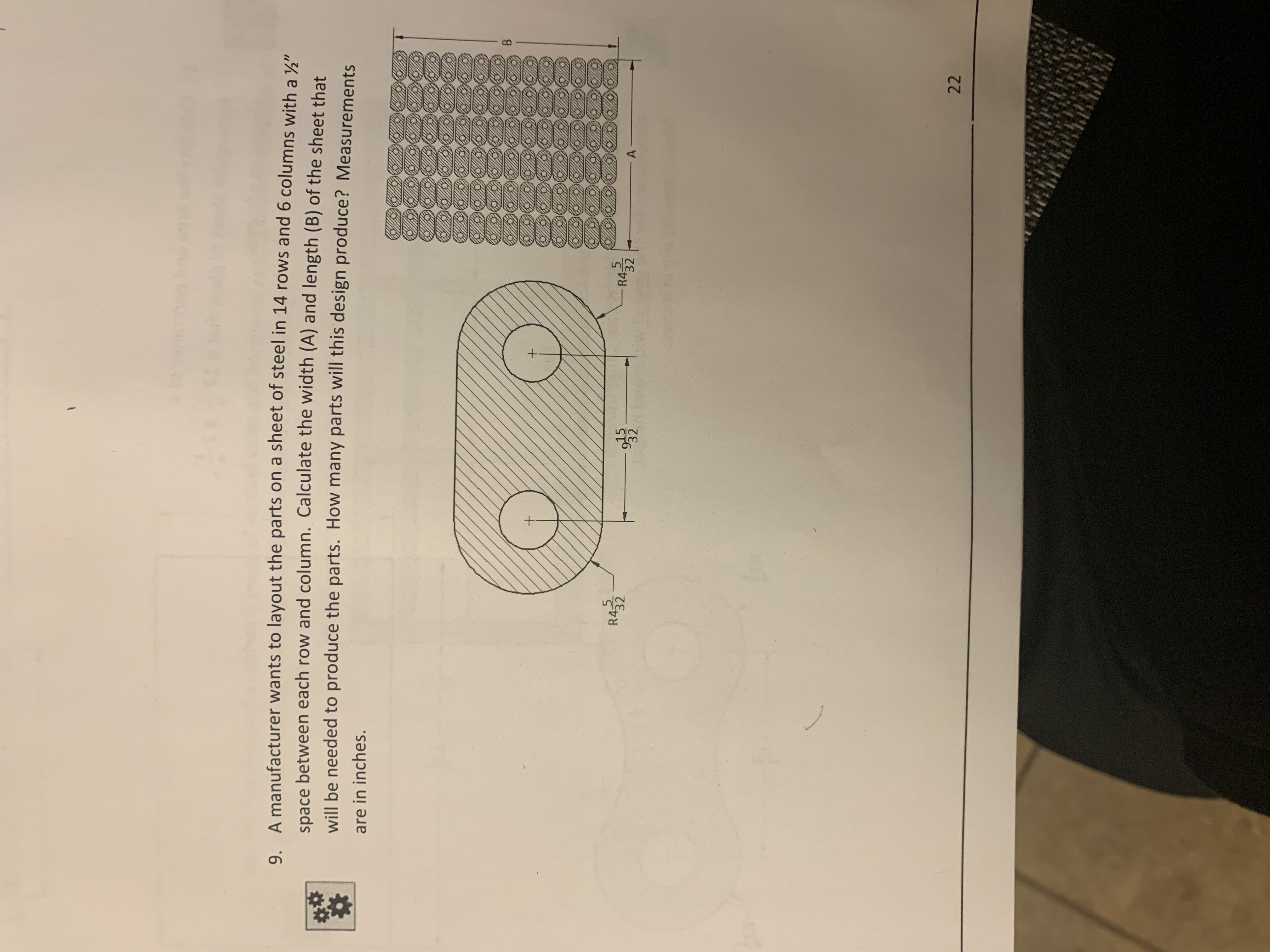# 9.A manufacturer wants to layout the parts on a sheet of steel in 14 rows and 6columns with aspace between each row and column. Calculate the width (A) and length (B) of the sheet that"will be needed to produe the parts. How many parts will this design produce? Measurementare in inchesR4-5R43232

Question
25 viewshelp_outlineImage Transcriptionclose9. A manufacturer wants to layout the parts on a sheet of steel in 14 rows and 6 columns with a space between each row and column. Calculate the width (A) and length (B) of the sheet that " will be needed to produe the parts. How many parts will this design produce? Measurement are in inches R4-5 R4 32 32 fullscreen
check_circle

Step 1

Given: 14-rows
6-columns
Space=1/2 between each row and column.

First, we calculate the width of the small section which is shown on the board, then after that we calculate the complete width A.

As the distance between the two-circle marked as positive sign is given as:

• 9(15/32) = 303/32inches

Now calculating the width of the left-over portion as the radius is given as:

• 4(5/32) = 133/32inches

This radius provided is the width of the one portion let it be the right-side potion and both are similar so we can twice our value. The radius so given is the width of the one portion.

Step 2

Total width of the left-over portion is 2 X 133/32 inches =266/32 inches.

Now its total width of this complete portion will be:

• 303/32inches +266/32 inches = 569/32 inches.

For calculating the whole width of the sheet in this length first we have two multiply it by 6 as it has columns and then we must add the space between each row and column.

• 6 X 569/32 inches =3414/32 inches

On the width we have 5 spaces in between the 6 columns and the length of one space is ½

Therefore, total space will be 5 X ½= 5/2 inches.

Step 3

Thus, the total width A will be sum of the total space between the row and column of the sheet and the total width of the section of the sheet = 3414...

### Want to see the full answer?

See Solution

#### Want to see this answer and more?

Solutions are written by subject experts who are available 24/7. Questions are typically answered within 1 hour.*

See Solution
*Response times may vary by subject and question.
Tagged in

### Math